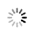# 在Pandas dataframe 中使用正则表达式创建新列

3月前 ⋅ 23 阅读

### ChatGPT的回答仅作参考：

```要在Pandas dataframe 中使用正则表达式创建新列，可以使用 `str.extract()` 方法。该方法可以从一个字符串列中提取匹配正则表达式的子字符串，并将其存储在新列中。

``` python
import pandas as pd

# 创建示例数据
data = {'email': ['john.doe@example.com', 'jane.doe@example.com', 'bob.smith@example.com']}
df = pd.DataFrame(data)

# 使用正则表达式提取用户名和域名
df['domain'] = df['email'].str.extract(r'@(\w+\.\w+)')

# 显示结果
print(df)
```

```
####全部评论: 0 条